# How to Find the Area of a Trapezium

How to Find the Area of a Trapezium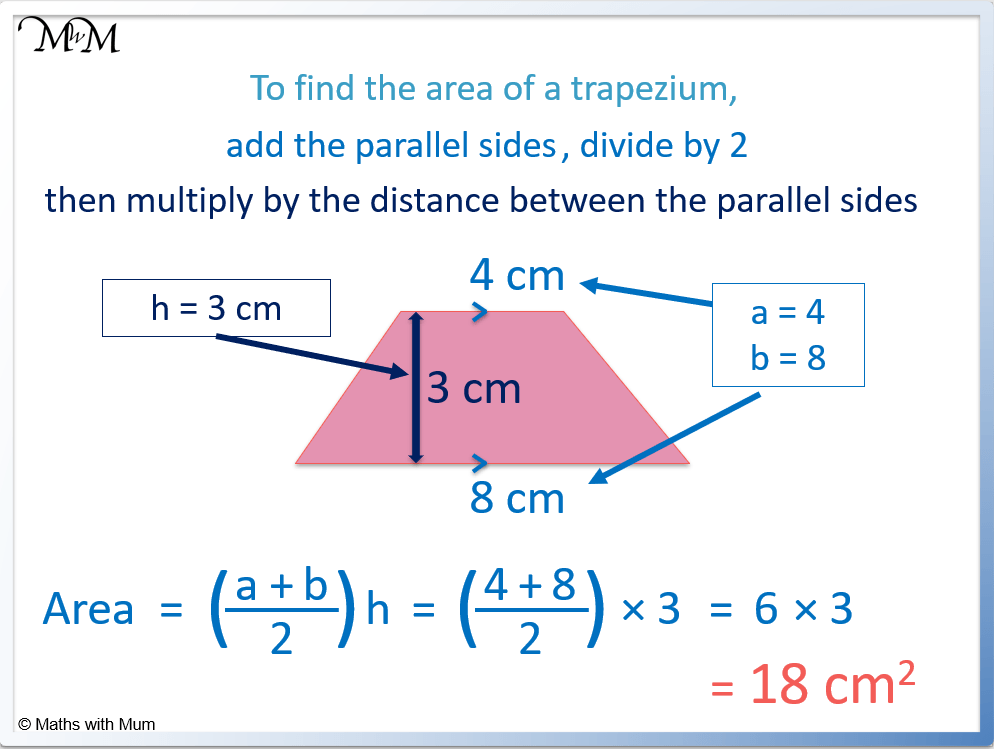• To find the area of a trapezium use the formula, $$Area = \frac{h}{2}(a+b)$$.
• a and b are the two parallel sides and h is the distance between them.
• In words, we add the two parallel sides, divide by 2 and multiply this by the distance between the parallel sides.
• Adding the parallel sides, 4 + 8 = 12. Dividing by 2, 12 ÷ 2 = 6. Multiplying by the distance between the parallel sides, 6 × 3 = 18.
• The units for area are squared, so the area is 18 cm2.

To find the area of a trapezium, add the parallel sides, divide by 2 and then multiply by the distance between the parallel sides.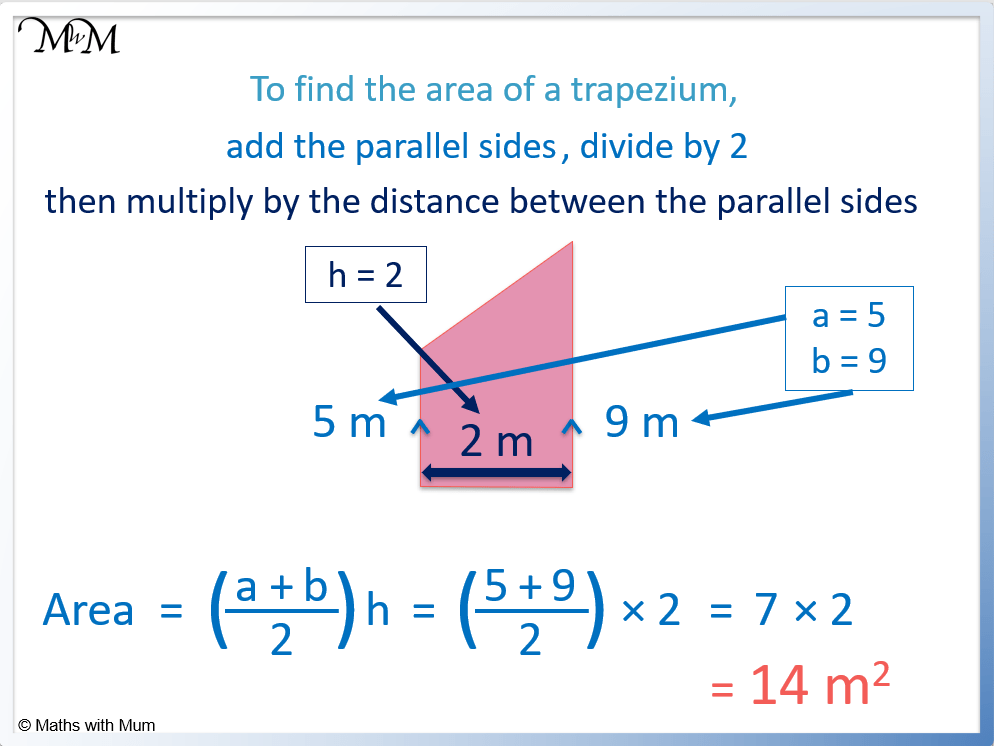• To find the area, add the parallel sides, divide by 2 and multiply by the distance between the parallel sides.
• The parallel sides are 5 and 9. Adding them, 5 + 9 = 14.
• Next divide by 2. 14 ÷ 2 = 7.
• Finally, multiply this by the distance between the parallel sides. 7 × 2 = 14.
• The area is 14 m2.
• Alternatively, use the formula of Area = h/2 (a + b), where a = 5, b = 9 and h =2.
• Area = h/2 (a + b) becomes Area = 2/2 (5 + 9) = 14.# Area of a Trapezium

## How to Find the Area of a Trapezoid

To find the area of a trapezoid:

1. Add the two parallel side lengths.
2. Multiply this by the distance between the parallel sides.
3. Divide this by 2.For example, find the area of the trapezoid with parallel side lengths 6m and 10m, with a distance of 4 m between them.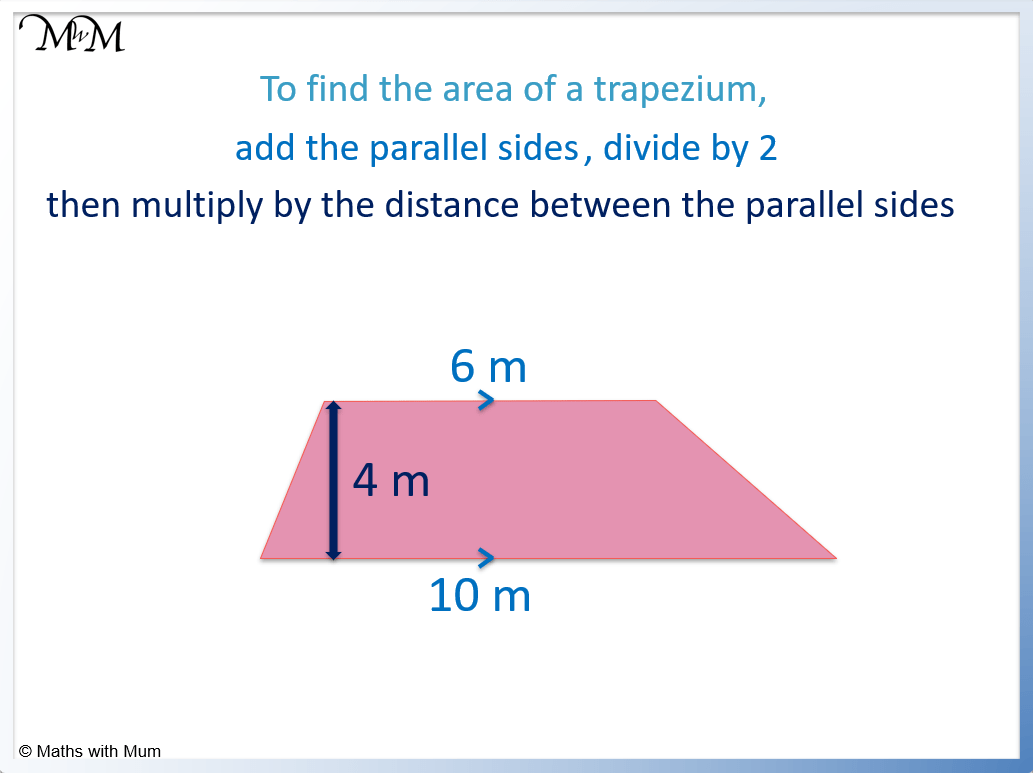1. Add the parallel sides: 6 + 10 = 16.
2. Multiply this by the distance between them: 16 × 4 = 64.
3. Divide by 2: 64 ÷ 2 = 32.

And so the area is 32 m2.

Alternatively, the area of a trapezoid can be found by using the formula Area = 1/2 (a+b)h, where a and b are the parallel sides of the trapezoid and h is the distance between them.

a = 6 and b = 10, while h = 4.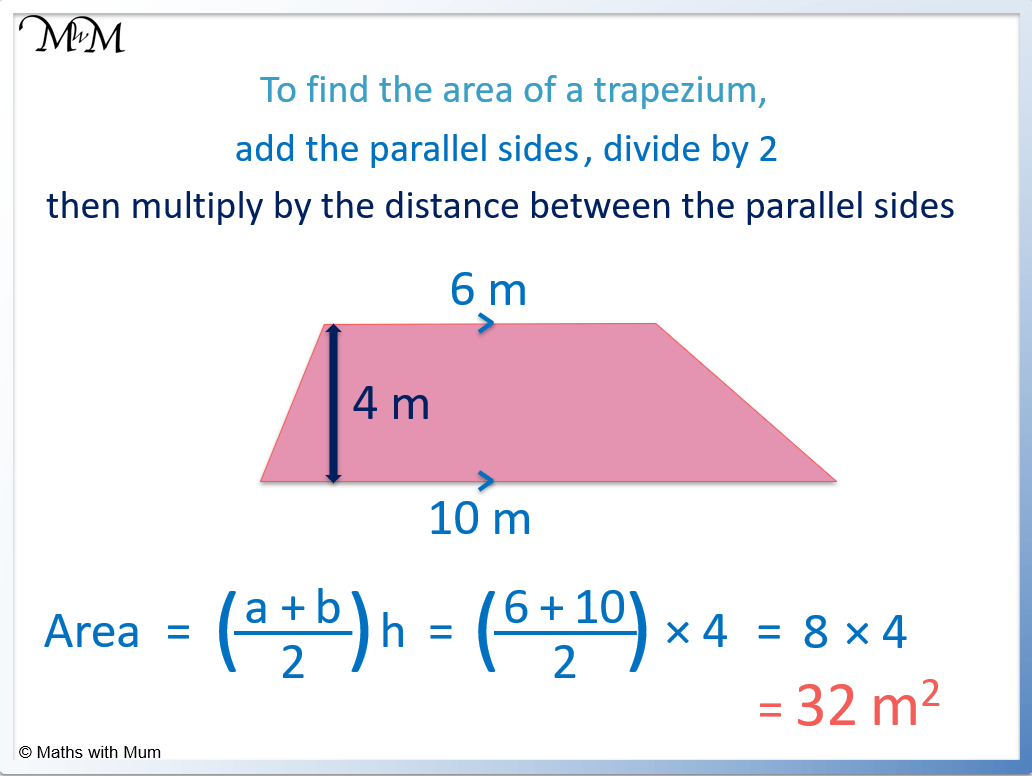Area = 1/2 (a+b)h becomes Area = 1/2 × (6 + 10) × 4.

This becomes Area = 1/2 × 16 × 4, which equals 32 m2.

## Formula for the Area of a Trapezoid

The formula for the area of a trapezoid is Area = h/2 (a+b), where a and b are the two parallel side lengths and h is the distance between them. For example, if a trapezoid has parallel sides of 5m and 7m and a distance between them of 4m, then a = 5, b = 7 and h = 4. The area formula becomes 4/2 (5+7) which equals 24 m2.

It does not matter which of the two parallel sides are labelled as a and b.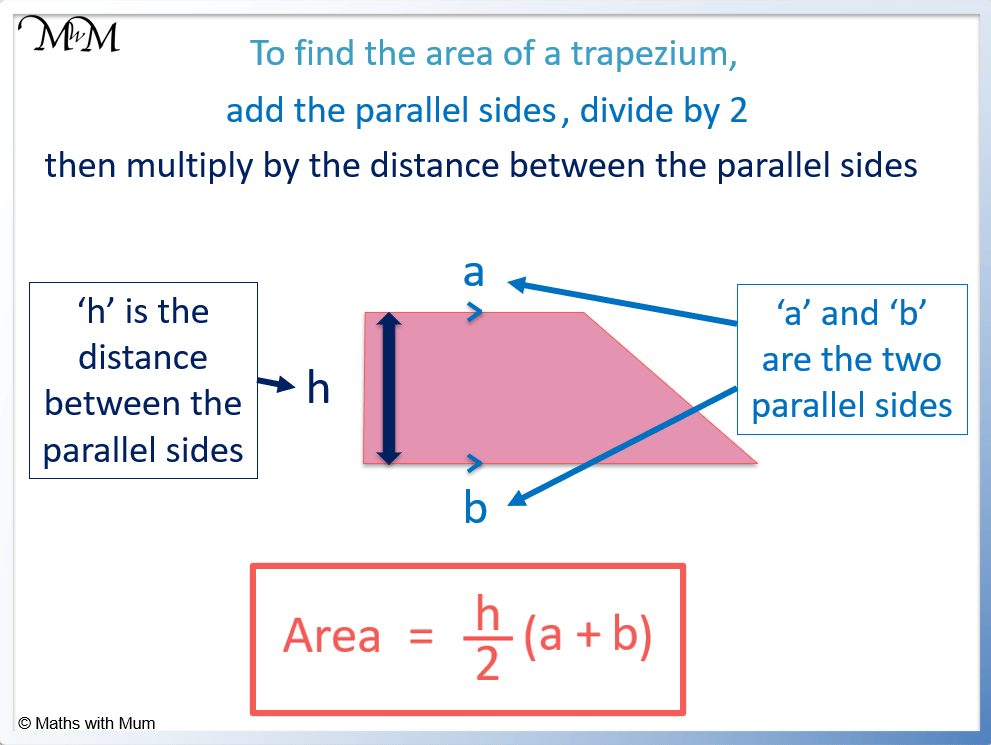The formula for the area of a trapezoid can also be written as Area = (a+b)/2 h.It can also be written as Area = 1/2 h(a+b) or Area = 1/2 (a+b)h.

All of these formula are just rearrangements of the same formula and will give the same answer.

## Area of a Right-Angled Trapezium

To find the area of a right-angled trapezoid, use the formula Area = 1/2 h(a+b), where a and b are the two parallel sides and h is the distance between them.

For example, calculate the area of the following right trapezoid.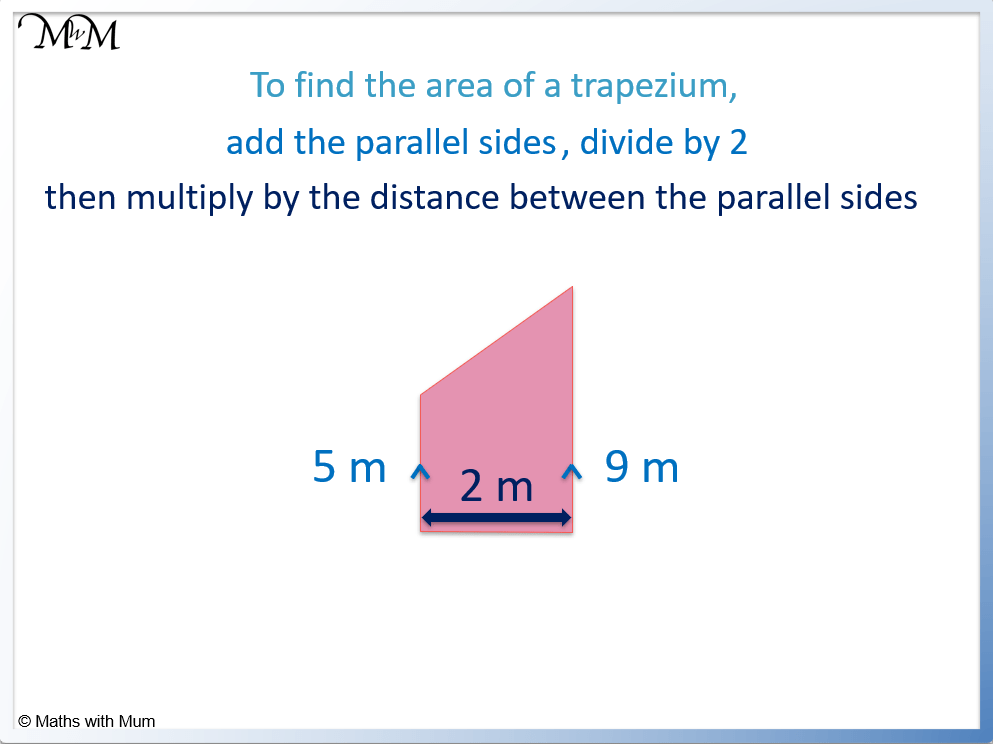The two parallel sides are 5 m and 9 m, so a = 5 and b = 9. It does not matter which side is a or which side is b.

The distance between the parallel sides is 2 m and so, h = 2.To find the area, substitue the values of h = 2, a = 5 and b = 9 into Area = 1/2 h(a+b).

Area = 1/2 h(a+b) becomes Area = 1/2 × 2 × (5 + 9)

This becomes Area = 1/2 × 2 × 14 which equals 14 m2.

## Area of an Isosceles Trapezoid

An isosceles trapezoid has diagonal sides that are the same length. These diagonal side lengths do not affect the area. To find the area of an isosceles trapezoid, use the formula Area = 1/2 h(a+b), where a and b are the lengths of the two parallel sides and h is the distance between them.

For example, here is an isosceles trapezoid. Its diagonal sides are the same size and it is symmetrical about its middle.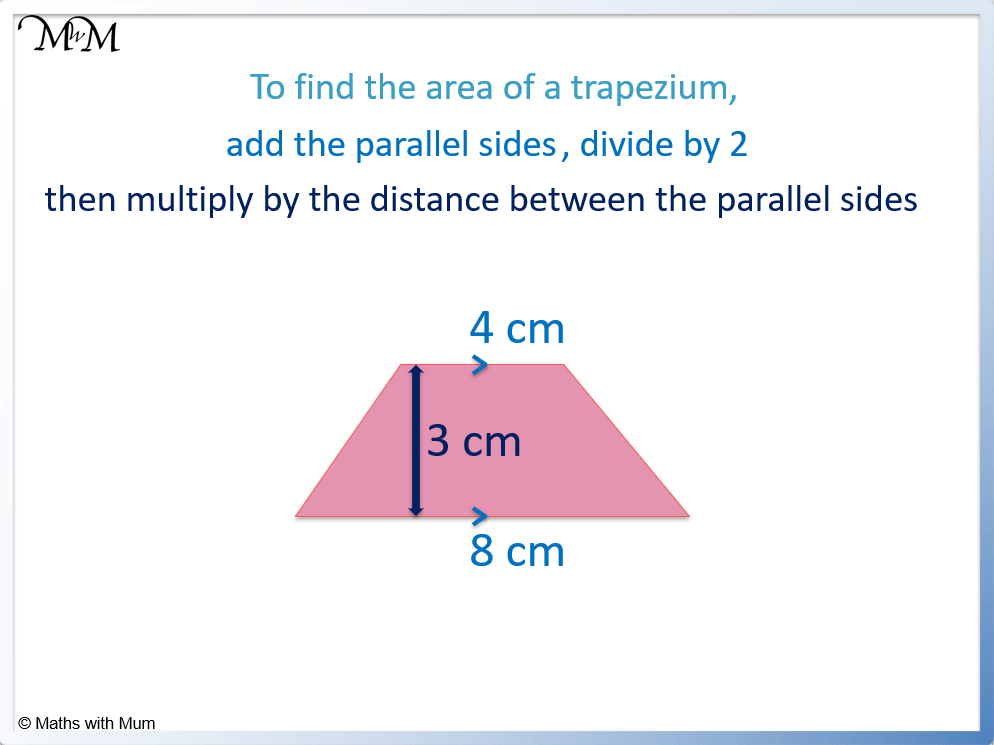The two parallel side lengths are 4 m and 8 m. So a = 4 and b = 8. It does not matter which side is a and which side is b out of these two values.

The distance between the parallel sides is 3 m. So h = 3.We substitute the values of h = 3, a = 4 and b = 8 into the formula Area = 1/2 h(a+b).

It becomes Area = 1/2 × 3 × (4 + 8), which becomes Area = 1/2 × 3 × 12.

It is easier to halve 12 than 3 so we halve the 12 to get 6.

Area = 3 × 6, which equals 18 cm2.

The area is measured in cm2 because the sides of the trapezoid are measured in cm.

## Area of an Irregular Trapezoid

The area of an irregular trapezoid is found in the usual manner using Area = 1/2 h(a+b). a and b are the two parallel sides and h is the distance between them. It does not matter what length the diagonal sides are in an irregular trapezoid as they do not affect the area.

For example, find the area of the irregular trapezoid shown.The two parallel sides are 6 m and 10 m. So a = 6 and b = 10. It does not matter which sides are a and b as long as they are parallel.

The distance between the parallel sides is 4 m, so h = 4.The area of the irregular trapezoid is found using the formula Area = 1/2 h(a+b).

Substituting in h = 4, a = 6 and b = 10, we get Area = 1/2 × 4 × (6 + 10).

This becomes Area = 1/2 × 4 × 16. Half of 16 is 8 and 8 × 4 = 32. The area is 32 m2.

## Area of a Trapezoid without the Height

If the height of a trapezoid is not given, it must be calculated using Pythagoras’ theorem. To do this, imagine a right-angled triangle formed on one side of the trapezoid, where the diagonal side is the hypotenuse. Square the hypotenuse, subtract the base of the triangle squared and then square root this to find the height of the trapezoid. The area can then be found using A = 1/2 h(a+b).

Here is an example of calculating the area of a trapezoid without knowing the height.The first step is to create a right-angled triangle on one side of the trapezoid. The hypotenuse of the triangle is the diagonal side of the trapezoid, in this case, it is 5 m.

Next, find the base of the triangle, which is the difference between the top and bottom side lengths of the trapezoid. 7 – 3 = 4 and so, the base of the triangle is 4 m.

The next step is to use Pythagoras’ theorem to work out the height of the triangle. This will be the same as the height of the trapezoid.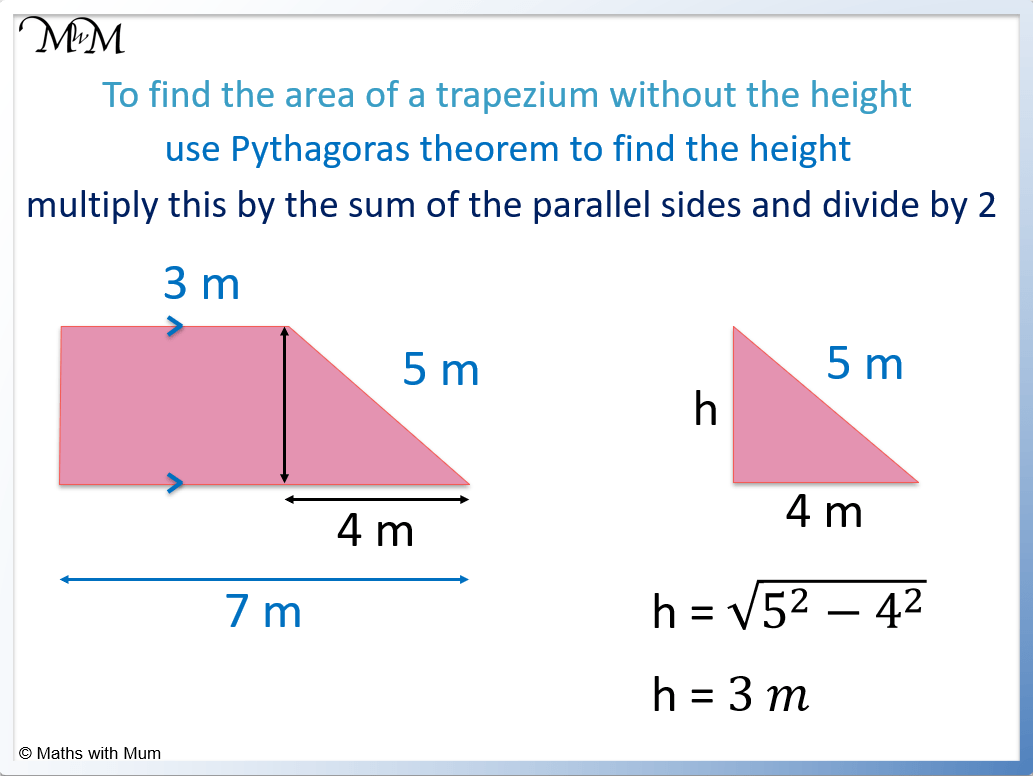Since the hypotenuse is known, the height of the triangle is one of the shorter sides of the triangle. To find the height of the triangle, use the formula: $$h = \sqrt{c^2 – b^2}$$ where h is the height, c is the diagonal side of the triangle and b is the base of the triangle.

52 – 42 = 9 and then the square root of 9 is 3. The height is 3.

Now the area of the trapezoid can be found using Area = 1/2 h(a+b).

h is the height of the trapezoid, b is the base of the trapezoid and a is the top side of the trapezoid.

Area = 1/2 × 3 × (3 + 7) = 152.

## Proof of the Area of a Trapezoid Formula

A trapezoid of height ‘h’, and parallel side lengths ‘a’ and ‘b’ can be made out of a triangle and a rectangle combined. The area of the rectangle is ah and the area of the triangle is 1/2 h(b-a). Adding the area of the triangle and rectangle together proves the formula for the area of a trapezoid, Area = 1/2 h(a+b).

The proof of the area of a trapezoid is shown below.

The trapezoid has height ‘h’, base ‘b’ and a top length of ‘a’. It can be split into two separate shapes, a rectangle and a triangle.

The rectangle area is length × width, which is a × h or ah. We call this Area 1, A1.

The triangle area is 1/2 × base × height. The base length of the triangle is the longer side of the trapezoid subtract the shorter side, b-a. The area of the triangle, A2 = 1/2 h(b-a).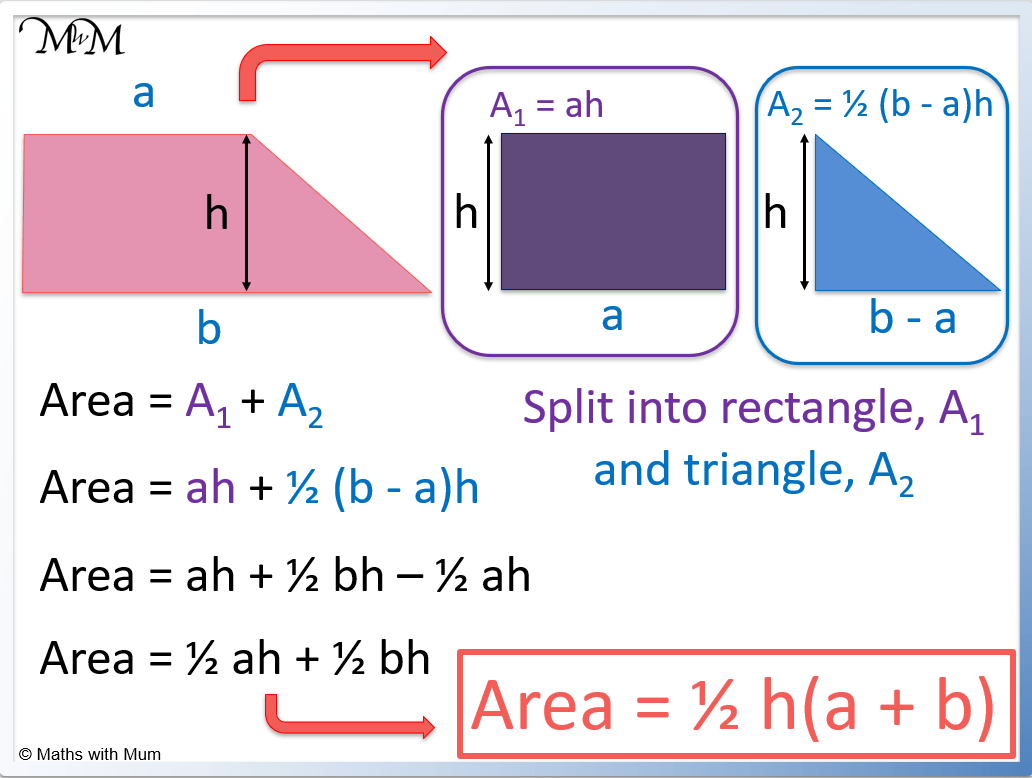We add the area of the rectangle to the area of the triangle to find the area of the trapezoid.

Area = A1 + A2.

Area = ah + 1/2 h(b-a).

Expanding the second term we get:

Area = ah + 1/2 bh – 1/2 ah.

Subtracting 1/2 ah from ah, we get:

Area = 1/2 ah + 1/2 bh.

Factorising the 1/2 and the h, we get the final area of a trapezoid:

Area = 1/2 h(a + b).

Here is an alternative proof of the area of a trapezoid in which we put two trapezoids together to form a rectangle.

Two identical trapezoids with height ‘h’, base ‘b’ and top side ‘a’ can be placed together to form a rectangle. This rectangle has height ‘h’ and a base of length ‘a+b’. The area of the rectangle is base × height, which is h × (a+b). The area of each individualy trapezoid is half of this, which is 1/2 h(a+b).The area of a rectangle is base × height. We can see that the height of the rectangle is the same as the height of each trapezium and the base of the rectangle is made up of the base of the trapezium and the top side of the trapezium combined. The base of the rectangle is a+b.

The area of the rectangle is h(a+b), however, each trapezoid is half of the total rectangle area because there are two of them. We halve the area of the rectangle to prove the area of the trapezoid.

Area = 1/2 h(a+b).Now try our lesson on Heron’s Formula for the Area of a Triangle with 3 Sides where we learn how to share an amount into a ratio of 3 parts.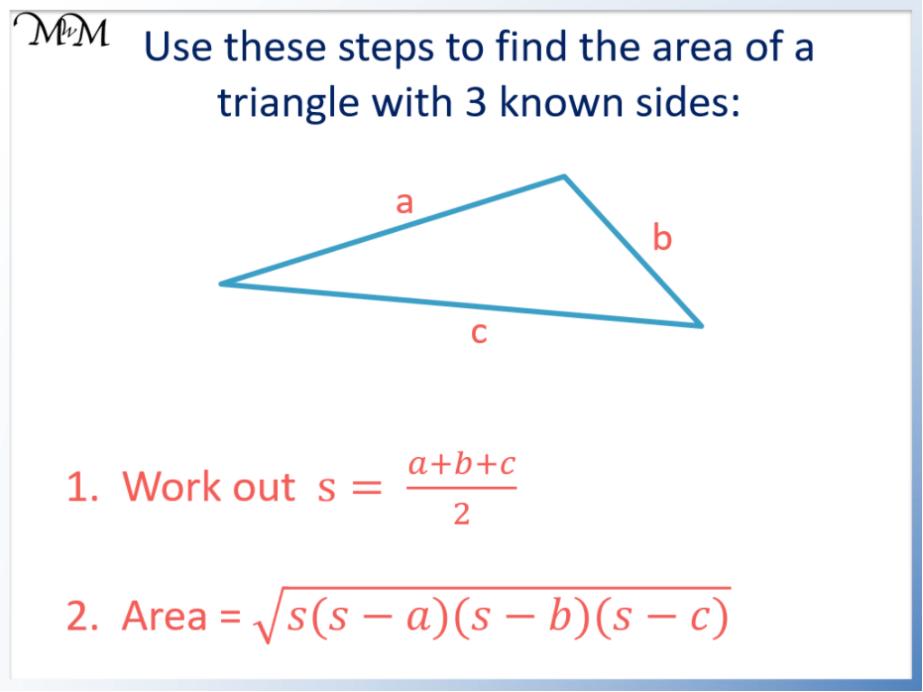error: Content is protected !!